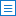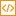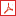Select Dynamics of momentum distribution and structure factor in a weakly interacting Bose gas with a periodical modulation Ning Liu(刘宁), Z C Tu(涂展春) Communications in Theoretical Physics    2020, 72 (12): 125501-.   DOI: 10.1088/1572-9494/abb7f0Abstract （58）HTML （1）PDF （386KB）（70）       The momentum distribution and dynamical structure factor in a weakly interacting Bose gas with a time-dependent periodic modulation in terms of the Bogoliubov treatment are investigated. The evolution equation related to the Bogoliubov weights happens to be a solvable Mathieu equation when the coupling strength is periodically modulated. An exact relation between the time derivatives of momentum distribution and dynamical structure factor is derived, which indicates that the single-particle property is strongly related to the two-body property in the evolutions of Bose-Einstein condensates. It is found that the momentum distribution and dynamical structure factor cannot display periodical behavior. For stable dynamics, some particular peaks in the curves of momentum distribution and dynamical structure factor appear synchronously, which is consistent with the derivative relation.
 Select Dynamic magnetic properties of Ising graphene-like monolayer Lei Sun, Wei Wang Communications in Theoretical Physics    2020, 72 (11): 115703-.   DOI: 10.1088/1572-9494/abb7d0Abstract （36）HTML （2）PDF （1006KB）（62）       Dynamic magnetic properties of the mixed-spin (3/2, 5/2) Ising graphene-like monolayer in an oscillating magnetic field are studied by means of Monte Carlo simulation. The effects of Hamiltonian parameters such as crystal field and time-dependent oscillating magnetic field on the dynamic order parameter, susceptibility and internal energy of the system are well presented and explained. Moreover, much attention has also been dedicated to the phase diagrams with different parameters in order to better comprehend the impacts of these parameters on the critical temperature. Our results reveal that the crystal fields of two sublattices have similar effects on the critical temperature, but the bias field and amplitude of oscillating field have opposite effects on it. We hope that our research can be of guiding significance to the theoretical and experimental studies of graphene-like monolayer.
 Select A note on the novel 4D Einstein–Gauss–Bonnet gravity Wen-Yuan Ai Communications in Theoretical Physics    2020, 72 (9): 95402-.   DOI: 10.1088/1572-9494/aba242Abstract （156）HTML （2）PDF （329KB）（123）       Recently, a novel 4D Einstein–Gauss–Bonnet gravity has been proposed by Glavan and Lin (2020 Phys. Rev. Lett. 124 081301) by rescaling the coupling $\alpha \to \alpha /(D-4)$ and taking the limit $D\to 4$ at the level of equations of motion. This prescription, though was shown to bring non-trivial effects for some spacetimes with particular symmetries, remains mysterious and calls for scrutiny. Indeed, there is no continuous way to take the limit $D\to 4$ in the higher D-dimensional equations of motion because the tensor indices depend on the spacetime dimension and behave discretely. On the other hand, if one works with 4D spacetime indices the contribution corresponding to the Gauss–Bonnet term vanishes identically in the equations of motion. A necessary condition (but may not be sufficient) for this procedure to work is that there is an embedding of the 4D spacetime into the higher D-dimensional spacetime so that the equations in the latter can be properly interpreted after taking the limit. In this note, working with 2D Einstein gravity, we show several subtleties when applying the method used in (2020 Phys. Rev. Lett. 124 081301).
 Select Quadratic, Higgs and hilltop potentials in Palatini gravity Nilay Bostan Communications in Theoretical Physics    2020, 72 (8): 85401-.   DOI: 10.1088/1572-9494/ab7ecbAbstract （37）HTML （1）PDF （1296KB）（56）       In this work, we study the theory of inflation with the non-minimally coupled quadratic, standard model Higgs, and hilltop potentials, through ξφ2R term in Palatini gravity. We first analyze observational parameters of the Palatini quadratic potential as functions of ξ for the high-N scenario. In addition to this, taking into account that the inflaton field φ has a non-zero vacuum expectation value v after inflation, we display observational parameters of well-known symmetry-breaking potentials. The types of potentials considered are the Higgs potential and its generalizations, namely hilltop potentials in the Palatini formalism for the high-N scenario and the low-N scenario. We calculate inflationary parameters for the Palatini Higgs potential as functions of v for different ξ values, where inflaton values are both φ > v and φ < v during inflation, as well as calculating observational parameters of the Palatini Higgs potential in the induced gravity limit for high-N scenario. We illustrate differences between the Higgs potential's effect on ξ versus hilltop potentials, which agree with the observations for the inflaton values for φ < v and ξ, in which v ≪ 1 for both these high and low N scenarios. For each considered potential, we also display ns − r values fitted to the current data given by the Keck Array/BICEP2 and Planck collaborations.
 Select Variational theory for the ground state and collective excitations of an elongated dipolar condensate P Blair Blakie, D Baillie, Sukla Pal Communications in Theoretical Physics    2020, 72 (8): 85501-.   DOI: 10.1088/1572-9494/ab95faAbstract （36）HTML （1）PDF （857KB）（45）       We develop a variational theory for a dipolar condensate in an elongated (cigar shaped) confinement potential. Our formulation provides an effective one-dimensional extended meanfield theory for the ground state and its collective excitations. We apply our theory to investigate the properties of rotons in the system comparing the variational treatment to a full numerical solution. We consider the effect of quantum fluctuations on the scattering length at which the roton excitation softens to zero energy.
 Select Aharonov–Bohm effect for bound states on spin-0 massive charged particles in a Gödel-type space–time with Coulomb potential Faizuddin Ahmed Communications in Theoretical Physics    2020, 72 (7): 75102-.   DOI: 10.1088/1572-9494/ab8a15Abstract （37）HTML （2）PDF （319KB）（58）       In this paper, we investigate the relativistic quantum dynamics of spin-0 massive charged particles in a Gödel-type space–time with electromagnetic interactions. We derive the radial wave equation of the Klein–Gordon equation with an internal magnetic flux field and Coulomb-type potential in the Som–Raychaudhuri space–time with cosmic string. We solve this equation and analyze the analog effect in relation to the Aharonov–Bohm effect for bound states.
 Select Hidden analytic relations for two-loop Higgs amplitudes in QCD Qingjun Jin, Gang Yang Communications in Theoretical Physics    2020, 72 (6): 65201-.   DOI: 10.1088/1572-9494/ab7ed8Abstract （69）HTML （4）PDF （715KB）（64）       We compute the Higgs plus two-quark and one-gluon amplitudes ($H\to q\bar{q}g$) and Higgs plus three-gluon amplitudes ($H\to 3g$) in the Higgs effective theory with a general class of operators. By changing the quadratic Casimir CF to CA, the maximally transcendental parts of the $H\to q\bar{q}g$ amplitudes turn out to be equivalent to that of the $H\to 3g$ amplitudes, which also coincide with the counterparts in ${ \mathcal N }=4$ SYM. This generalizes the so-called maximal transcendentality principle to the Higgs amplitudes with external quark states, thus the full QCD theory. We further verify that the correspondence applies also to two-loop form factors of more general operators, in both QCD and scalar-YM theory. Another interesting relation is also observed between the planar $H\to q\bar{q}g$ amplitudes and the minimal density form factors in ${ \mathcal N }=4$ SYM.
 Select Effect of confined one-gluon-exchange potential and instanton-induced interaction on nucleon–nucleon interaction S Raghavendra, C Vanamali Shastry, V K Nilakanthan, K B Vijaya Kumar Communications in Theoretical Physics    2020, 72 (6): 65301-.   DOI: 10.1088/1572-9494/ab8930Abstract （22）HTML （2）PDF （369KB）（73）       The effect of confined one-gluon-exchange potential and instanton-induced interaction potential in the singlet (1S0) and triplet (3S1) channels for nucleon–nucleon interaction has been investigated in the framework of the relativistic harmonic model using the resonating group method in the adiabatic limit with the Born–Oppenheimer approximation. The contributions of the different components of the interaction potentials have been analyzed.
 Select The fractional features of a harmonic oscillator with position-dependent mass Dumitru Baleanu, Amin Jajarmi, Samaneh Sadat Sajjadi, Jihad H Asad Communications in Theoretical Physics    2020, 72 (5): 55002-.   DOI: 10.1088/1572-9494/ab7700Abstract （92）HTML （1）PDF （716KB）（231）       In this study, a harmonic oscillator with position-dependent mass is investigated. Firstly, as an introduction, we give a full description of the system by constructing its classical Lagrangian; thereupon, we derive the related classical equations of motion such as the classical Euler–Lagrange equations. Secondly, we fractionalize the classical Lagrangian of the system, and then we obtain the corresponding fractional Euler–Lagrange equations (FELEs). As a final step, we give the numerical simulations corresponding to the FELEs within different fractional operators. Numerical results based on the Caputo and the Atangana-Baleanu-Caputo (ABC) fractional derivatives are given to verify the theoretical analysis.
 Select Perspectives on relativistic quantum chaos Liang Huang, Ying-Cheng Lai Communications in Theoretical Physics    2020, 72 (4): 47601-.   DOI: 10.1088/1572-9494/ab6909Abstract （171）HTML （2）PDF （2230KB）（292）       Quantum Chaos has been investigated for about a half century. It is an old yet vigorous interdisciplinary field with new concepts and interesting topics emerging constantly. Recent years have witnessed a growing interest in quantum chaos in relativistic quantum systems, leading to the still developing field of relativistic quantum chaos. The purpose of this paper is not to provide a thorough review of this area, but rather to outline the basics and introduce the key concepts and methods in a concise way. A few representative topics are discussed, which may help the readers to quickly grasp the essentials of relativistic quantum chaos. A brief overview of the general topics in quantum chaos has also been provided with rich references.
 Select Quantum information and microscopic measuring instruments Alexey V Melkikh Communications in Theoretical Physics    2020, 72 (1): 15101-.   DOI: 10.1088/1572-9494/ab5453Abstract （33）HTML （1）PDF （626KB）（94）       The consideration of measuring instruments as macroscopic bodies leads to neglect of the microscopic processes that occur during measurements. This disregard is not justified in general cases. As an example of measurements using microscopic instruments, the scattering of a photon by an electron with electron interference at two slits (Compton effect) was used. The amount of information that can be obtained in such a process is inversely proportional to the wavelength of the incident photon. At large photon wavelengths (soft measurements), the pure state of the electron can be disrupted by an arbitrarily small extent; accordingly, the amount of information extracted in such an experiment is also arbitrarily small. It is shown that the energy price for a bit obtained in such a measurement tends toward a constant value for increasing the photon wavelength. Microscopic instruments can be used in situations where energy costs for measurements are important.